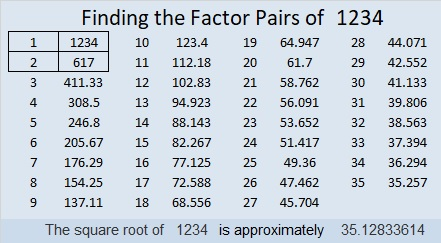# 1234 and Level 2

This is my 1234th post, so today’s puzzle has been given that number. Whenever I see 12:34 on a clock, I always think about my husband’s Uncle Paul who really liked noticing that time because all possible clock digits are used and the digits are in order. I also like those digits because 12 = 3 × 4.Print the puzzles or type the solution in this excel file: 12 factors 1232-1241

Here are some facts about the number 1234 some of which might surprise you:

• 1234 is a composite number.
• Prime factorization: 1234 = 2 × 617
• The exponents in the prime factorization are 1 and 1. Adding one to each and multiplying we get (1 + 1)(1 + 1) = 2 × 2 = 4. Therefore 1234 has exactly 4 factors.
• Factors of 1234: 1, 2, 617, 1234
• Factor pairs: 1234 = 1 × 1234 or 2 × 617
• 1234 has no square factors that allow its square root to be simplified. √1234 ≈ 35.128341234 is the sum of two squares:
35² + 3² = 1234

1234 is the hypotenuse of a Pythagorean triple:
210-1216-1234 calculated from 2(35)(3), 35² – 3², 35² + 3²
It is also times (105-608-617)